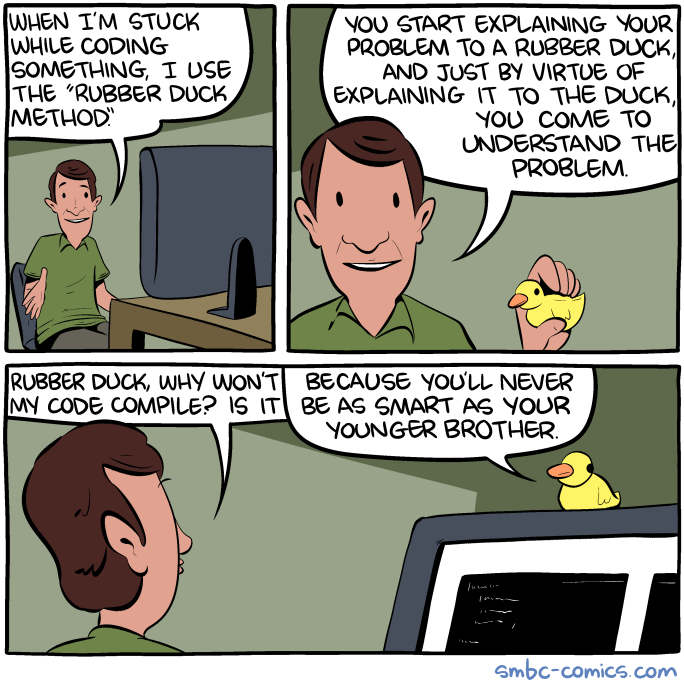A Short Debugging Guide¶

We have put together a list of common traps you may fall into, hopefully you will find the tools here to get yourself out of them!

• Did you forget conda activate sharpy_env and source bin/sharpy_vars.sh?

• If you do in the terminal: which sharpy, do you get the one you want?

• If you do which python, does the result point to anaconda3/envs/sharpy_env/bin (or similar)?

• Wrong input (inconsistent connectivities, mass = 0…)

• Sometimes not easy to detect. For the structural model, run BeamLoader and BeamPlot with no structural solver in between. Go over the structure in Paraview. Check the fem.h5 file with HDFView.

• Remember that connectivities are ordered as $[0, 2, 1]$ (the central node goes last).

• Make sure the num_elem and num_node variables are actually your correct number of elements and nodes.

• Not running the actual case you want to.

• Cleanup the folder and regenerate the case

• Not running the SHARPy version you want.

• Check at the beginning of the execution the path to the SHARPy folder.

• Not running the correct branch of the code.

• You probably want to use develop. Again, check the first few lines of SHARPy output.

• Very different (I’m talking orders of magnitude) stiffnesses between nodes or directions?

• Maybe the UVLM requires a smaller a smaller vortex core cutoff (only for linear UVLM simulations, as the nonlinear uses another vortex core model).

• Newmark damping is not enough for this case?

• Do you have an element with almost 0 mass or inertia?

• Are you mass matrices consistent? Check that $$I_{xx} = I_{yy} + I_{zz}$$.

• Have a look at the $$\dot{\Gamma}$$ filtering and numerical parameters in the settings of StepUvlm and DynamicCoupled.

• Add more relaxation to the StaticCoupled or DynamicCoupled solvers.

• The code has a bug (depending on where, it may be likely).

• Go over the rest of the list. Plot the case in paraview. Go over the rest of the list again. Prepare the simplest example that reproduces the problem and raise an issue.

• The code diverges because it has to (physical unstable behaviour)
• Then don’t complain

• Your model still doesn’t work and you don’t know why.
• import pdb; pdb.set_trace() and patience

• If nothing else works… get a rubber duck (or a very very patient good friend) and go over every stepIf your model doesn’t do what it is supposed to do:

• Check for symmetric response where the model is symmetric.

• If it is not, run the beam solver first and make sure your properties are correct. Make sure the matrices for mass and stiffness are rotated if they need to be (remember the Material FoR definition and the for_delta?)

• Now run the aerodynamic solver only and double check that the forces are symmetric.

• Make sure your tolerances are low enough so that at least 4 FSI iterations are performed in StaticCoupled or DynamicCoupled.

• Make sure your inputs are correct. For example: a dynamic case can be run with $$u_\infty = 0$$ and the plane moving forwards, or $$u_\infty = x$$ whatever and the plane velocity = 0. It is very easy to mix both, and end up with double the effective incoming speed (or none).

• Run simple stuff before coupling it. For example, if your wing tip deflections don’t match what you’d expect, calculate the deflection under a small tip force (not too small, make sure the deflection is > 1% of the length!) by hand, and compare.

• It is more difficult to do the same with the UVLM, as you need a VERY VERY high aspect ratio to get close to the 2D potential solutions. You are going to have to take my word for it: the UVLM works.

• But check the aero grid geometry in Paraview, including chords lengths and angles.